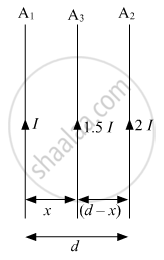# Answer the Following Question. Two Infinitely Long Straight Wire A1 And A2 Carrying Currents I and 2i Flowing in the Same Direction Are Kept' Distance Apart. - Physics

Numerical

Two infinitely long straight wire A1 and A2 carrying currents I and 2I flowing in the same direction are kept' distance apart. Where should a third straight wire A3 carrying current 1.5 I be placed between A1 and A2 so that it experiences no net force due to A1 and A2? Does the net force acting on A3 depend on the current flowing through it?

#### Solution"F"_31 = "F"_32

("μ"_0("I") (1.5 "I"))/(4"π"(x)) = ("μ"_0 (1.5 "I") (2 "I"))/(4"π"(d - x))

(1)/(x) = (1)/(d - x)

d - x = x

d = 2x

x = d/(2)

The net force acting on A3,
|"F"_"net"| = |("μ"_0("I") ("I"_("A"3)))/(4"π"(x)) = ("μ"_0("I"_("A"3)) (2 "I"))/(4"π"(d -x))|
Hence, it depends on the value of the current flowing through A3.

Concept: Force Between Two Parallel Currents, the Ampere
Is there an error in this question or solution?